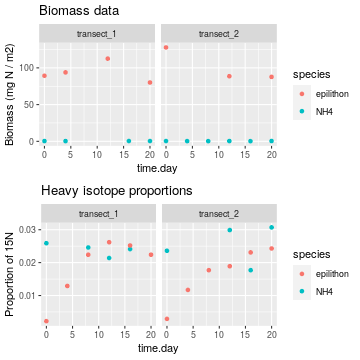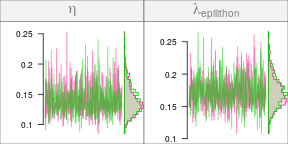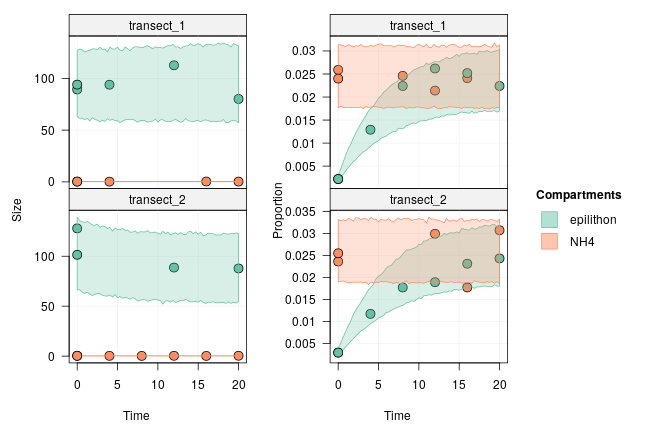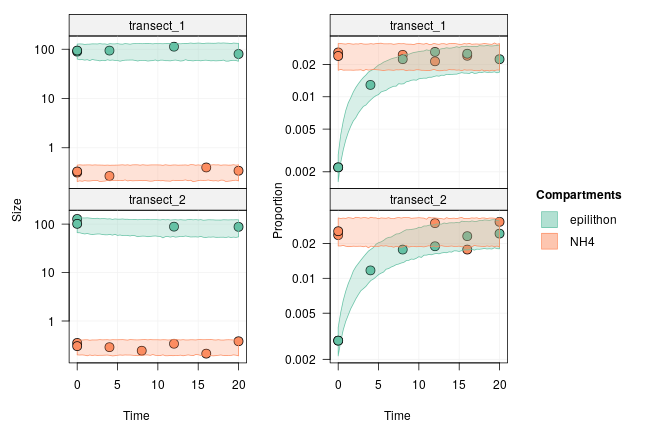#### 2021-09-24

In some experiments, you might want to specify that some compartments are in a steady-state: their size and tracer content will not change due to nutrient flows from or into other compartments. This is equivalent to having an infinitely buffered compartment, and it is useful to model some real-life situations such as:

• The dissolved inorganic nutrients in a stream reach: when performing addition experiments in a stream, the water flow constantly renews the pool of dissolved inorganic nutrients over the experiment location, and those inorganic nutrient pools can often be considered in a steady state at the temporal and spatial scale of the experiment.

• The source nutrients when in very large excess compared to the rest of the network compartments: for example, when performing experiments with a few insect individuals feeding on Petri dishes containing the enriched nutrients, the nutrient sources can be so abundant compared to the biomass consumed by the insects that a steady state can be a good approximation for those compartments.

In this tutorial, we will learn how to define a compartment as being in a steady state in a network model.

library(isotracer)
library(tidyverse)

## Data preparation

The simulated data we use in this example can be loaded into your R session by running the code below:

exp <- tibble::tribble(
~time.day,    ~species, ~biomass, ~prop15N,    ~transect,
0,       "NH4",    0.313,   0.0259, "transect_1",
4,       "NH4",   0.2675,       NA, "transect_1",
8,       "NH4",       NA,   0.0246, "transect_1",
12,       "NH4",       NA,   0.0214, "transect_1",
16,       "NH4",   0.3981,   0.0241, "transect_1",
20,       "NH4",   0.3414,       NA, "transect_1",
0, "epilithon",  89.2501,   0.0022, "transect_1",
4, "epilithon",  93.8583,   0.0129, "transect_1",
8, "epilithon",       NA,   0.0224, "transect_1",
12, "epilithon", 112.5986,   0.0262, "transect_1",
16, "epilithon",       NA,   0.0252, "transect_1",
20, "epilithon",  80.0911,   0.0224, "transect_1",
0,       "NH4",   0.3525,   0.0236, "transect_2",
4,       "NH4",   0.2881,       NA, "transect_2",
8,       "NH4",   0.2436,       NA, "transect_2",
12,       "NH4",   0.3392,   0.0299, "transect_2",
16,       "NH4",    0.212,   0.0177, "transect_2",
20,       "NH4",   0.3818,   0.0307, "transect_2",
0, "epilithon",  127.873,   0.0029, "transect_2",
4, "epilithon",       NA,   0.0117, "transect_2",
8, "epilithon",       NA,   0.0177, "transect_2",
12, "epilithon",  88.6496,   0.0189, "transect_2",
16, "epilithon",       NA,   0.0231, "transect_2",
20, "epilithon",  87.7534,   0.0243, "transect_2"
)

The simulated experiment is taking place in a stream at two locations (transect_1 and transect_2). The network we want to model has two compartments: the ammonium NH$$_4^+$$ dissolved in the stream water and the algae growing on the stream bed (epilithon) which can assimilate ammonium from the water.

Let's have a look at the data:

library(ggplot2)
library(gridExtra)
p1 <- ggplot(exp, aes(x = time.day, y = biomass, col = species)) +
geom_point() + ggtitle("Biomass data") + ylab("Biomass (mg N / m2)") +
facet_wrap(~ transect)
p2 <- ggplot(exp, aes(x = time.day, y = prop15N, col = species)) +
geom_point() + ggtitle("Heavy isotope proportions") + ylab("Proportion of 15N")  +
facet_wrap(~ transect)
grid.arrange(p1, p2, nrow = 2)Because the water is constantly flowing in the stream, we will consider that ammonium is in a steady state at the local scale of a transect (i.e. whatever is consumed by epilithon is replenished by the incoming water). The dissolved ammonium is experimentally enriched in $$^{15}$$N compared to background levels by dripping a solution of $$^{15}$$N-enriched ammonium at a constant rate upstream of each transect (the dripping starts at the beginning of the experiment). This is why the proportion of $$^{15}$$N in ammonium is higher than in epilithon at the beginning of the experiment.

As long as the drip is also stable during the experiment, the assumption of steady state for dissolved ammonium holds. We will learn in the next tutorial how we can specify more complicated addition events, such as pulses or on/off drip regimes.

## Building the network model

Let's start by creating a new network model and specifying its topology:

m <- new_networkModel() %>% set_topo("NH4 -> epilithon")

The next step is to define the initial conditions. In our simulated experiment, the data comes from measurements in a stream, not from a controlled aquarium, so the initial conditions are not as certain as in a controlled experiment. We will assume that a good guess for initial biomasses are the mean biomasses measured in each transect:

init_bm <- exp %>%
group_by(species, transect) %>%
summarize(biomass = mean(biomass, na.rm = TRUE))
## summarise() has grouped output by 'species'. You can override using the .groups argument.
init_bm
## # A tibble: 4 × 3
## # Groups:   species 
##   species   transect   biomass
##   <chr>     <chr>        <dbl>
## 1 epilithon transect_1  93.9
## 2 epilithon transect_2 101.
## 3 NH4       transect_1   0.33
## 4 NH4       transect_2   0.303

For the initial proportion of $$^{15}$$N in the dissolved ammonium, we will also use mean values since we expect ammonium to be in a steady state during the experiment (the drip is on during the whole experiment):

init_prop_NH4 <- exp %>%
filter(species == "NH4") %>%
group_by(transect, species) %>%
summarize(prop15N = mean(prop15N, na.rm = TRUE))
## summarise() has grouped output by 'transect'. You can override using the .groups argument.
init_prop_NH4
## # A tibble: 2 × 3
## # Groups:   transect 
##   transect   species prop15N
##   <chr>      <chr>     <dbl>
## 1 transect_1 NH4      0.024
## 2 transect_2 NH4      0.0255

For the epilithon, we know that the proportion of $$^{15}$$N will increase during the experiment, so our best guess is to use only the proportion measured at $$t_0$$:

init_prop_epi <- exp %>%
filter(species == "epilithon" & time.day == 0) %>%
select(species, prop15N, transect)
init_prop_epi
## # A tibble: 2 × 3
##   species   prop15N transect
##   <chr>       <dbl> <chr>
## 1 epilithon  0.0022 transect_1
## 2 epilithon  0.0029 transect_2

Now we can wrap up all those numbers into a single table containing the initial conditions:

init_prop <- bind_rows(init_prop_NH4, init_prop_epi)
inits <- full_join(init_bm, init_prop)
inits
## # A tibble: 4 × 4
## # Groups:   species 
##   species   transect   biomass prop15N
##   <chr>     <chr>        <dbl>   <dbl>
## 1 epilithon transect_1  93.9    0.0022
## 2 epilithon transect_2 101.     0.0029
## 3 NH4       transect_1   0.33   0.024
## 4 NH4       transect_2   0.303  0.0255

We can use this table to specify the initial conditions of the model:

m <- m %>% set_init(inits, comp = "species", size = "biomass", prop = "prop15N",
group_by = "transect")

We finally add all the observations:

m <- m %>% set_obs(exp, time = "time.day")
m
## # A tibble: 2 × 5
##   topology           initial          observations      parameters       group
##   <list>             <list>           <list>            <list>           <list>
## 1 <topology [2 × 2]> <tibble [2 × 3]> <tibble [12 × 4]> <tibble [5 × 2]> <chr >
## 2 <topology [2 × 2]> <tibble [2 × 3]> <tibble [12 × 4]> <tibble [5 × 2]> <chr >

Let's check the grouping structure of our model:

groups(m)
## # A tibble: 2 × 1
##   transect
##   <chr>
## 1 transect_1
## 2 transect_2

Good!

The last step before we can run the MCMC is to specify that the NH$$_4^+$$ compartment is in steady state. Let's have a look at the current topology of the model:

topo(m)
## <2 comps>
##           epilithon NH4
## epilithon         0   1
## NH4               0   0

All compartments in the current topology are "standard" compartments (i.e. not in imposed steady-state). We use the set_steady() function to indicate which compartments are to be considered into steady-state:

m <- m %>% set_steady(comps = "NH4")

NH4 is set to steady-state: the compartment size and the proportion of $$^{15}$$N for NH4 will be considered constant within each replicate. Let's look again at the topology:

topo(m)
## <2 comps>
##           epilithon NH4*
## epilithon         0    1
## NH4*              0    0
## [ * : steady-state]

NH$$_4^+$$ is now marked with an asterisk, which indicates a steady state compartment. We are ready to run the MCMC.

## Running the MCMC

As usual, let's have a look at the parameters which are going to be fit and at their priors:

priors(m)
## # A tibble: 5 × 2
##   in_model                 prior
##   <chr>                    <list>
## 1 eta                      <hcauchy (scale=0.1)>
## 2 lambda_epilithon         <hcauchy (scale=0.1)>
## 3 lambda_NH4               <hcauchy (scale=0.1)>
## 4 upsilon_NH4_to_epilithon <hcauchy (scale=0.1)>
## 5 zeta                     <hcauchy (scale=0.1)>

Note that since NH$$_4^+$$ is in a steady state in the model, the lambda_NH4 parameter will not influence the likelihood of the model, and the posterior for this parameter will basically be its prior distribution.

We keep the default priros and run the model:

run <- run_mcmc(m, iter = 1000)
plot(run)
# Note: the figure below only shows a few of the traceplots for vignette concisionThe traces look good. Let's do a posterior predictive check to ensure that the model makes sense:

predictions <- predict(m, run)
plot(predictions, facet_row = "group")Let's use a log scale on the y axis to make visualization of the two compartments in the same plots easier:

plot(predictions, facet_row = "group", log = TRUE)The model looks ok.

In the next tutorial, you will learn how to specify more complex addition regimes using pulse and drip events.